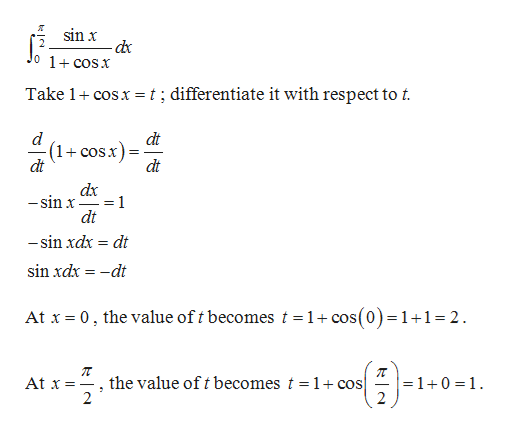In solving the integration of: (sin x) / (1+ cos x)  [0, pi/2] where u= (1+ cos x) and du = -sin x dx, the solution converted the interval of [0, pi/2] to [2, 1].       I don't understand why this was necessary since the order of the intervals seem reversed. Could you give me a step by step explanation why this was necessary?

Question

In solving the integration of: (sin x) / (1+ cos x)  [0, pi/2] where u= (1+ cos x) and du = -sin x dx, the solution converted the interval of [0, pi/2] to [2, 1].       I don't understand why this was necessary since the order of the intervals seem reversed. Could you give me a step by step explanation why this was necessary?

Step 1

The function is

Step 2

To compute:help_outlineImage Transcriptioncloseя sin x d 0 1+cosX Take 1 cosx= t; differentiate it with respect to t. d (1cosx) dt dt dt dx 1 -sin x dt -sin xdx dt sin xdx dt At x 0, the value of t becomes t =1+ cos(0)=1+1= 2 п the value oft becomes t = 1 +cos] 2 At x - =1+0 1 - fullscreen
Step 3

Thus, the definite integral with...

Want to see the full answer?

See Solution

Want to see this answer and more?

Our solutions are written by experts, many with advanced degrees, and available 24/7

See Solution
Tagged in

Calculus